# Functions for lookup of data

These functions perform a lookup on a data range using the provided value and return the result.

Returns the cell reference for the given row and column, or the current cell.

If you do not specify a row and column, the current cell is used.

Example:

#### CELL

Returns the type of value in the given data. Similar to the TYPE function.

CELL(data)

The function can return these values:
array   = a data array or cell range
blank   = the following values are considered blank: absent data, empty string "", a single space " ", a "0" text, and a 0 number
error   = a formula error
period  = a period YYYY-MM
date    = a date YYYY-MM-DD
number  = a numerical value
boolean = a boolean value
phone   = a phone number
url     = a website URL
wrap    = a JSON-formatted text (see WRAP function)
text    = a text string

Example:
CELL(123) = "number"
CELL(A3) = "text"

#### CELLRANGE ❖ XLReporting

Returns an array with the given cell values, arrays or cell ranges. Using INDEX you can extract individual values from the array. You may specific multiple ranges.

CELLRANGE(data, data, ..)

Example:
CELLRANGE(A1:A3, B5, C10:C12)

#### CELLS

Returns the number of cells in the given cell range or data array.

CELLS(data)

Example:
CELLS(A1:C4) = 12

#### CELLVALUE ❖ XLReporting

Returns the value in the cell with the given cell reference. You can optionally provide the sheet name (as text), else the default sheet (the first or only sheet in the model) will be used.

This is only relevant in models and enables you to obtain the value in a cell by passing a dynamic text reference to that cell. This enables you to dynamically calculate the reference to a cell within a formula without having to change the formula itself.

IMPORTANT: this function requires that the cell reference and sheet name is given as quoted text strings, for example CELLVALUE("A1", "Sheet1"). This is intentionally different from most other functions because CELLVALUE refers to a cell by means of a text reference.

Please be aware that sheet and cell references made via CELLVALUE are not adjusted when sheets are renamed and/or rows or columns are inserted or deleted.

CELLVALUE(cell, sheet)

Example:
CELLVALUE("C2") = 25
CELLVALUE("C2", "Sheet1") = 25

#### CHOOSE

Returns the value of the given index in the given cell range, data array, or comma-delimited list of values.

CHOOSE(data, index)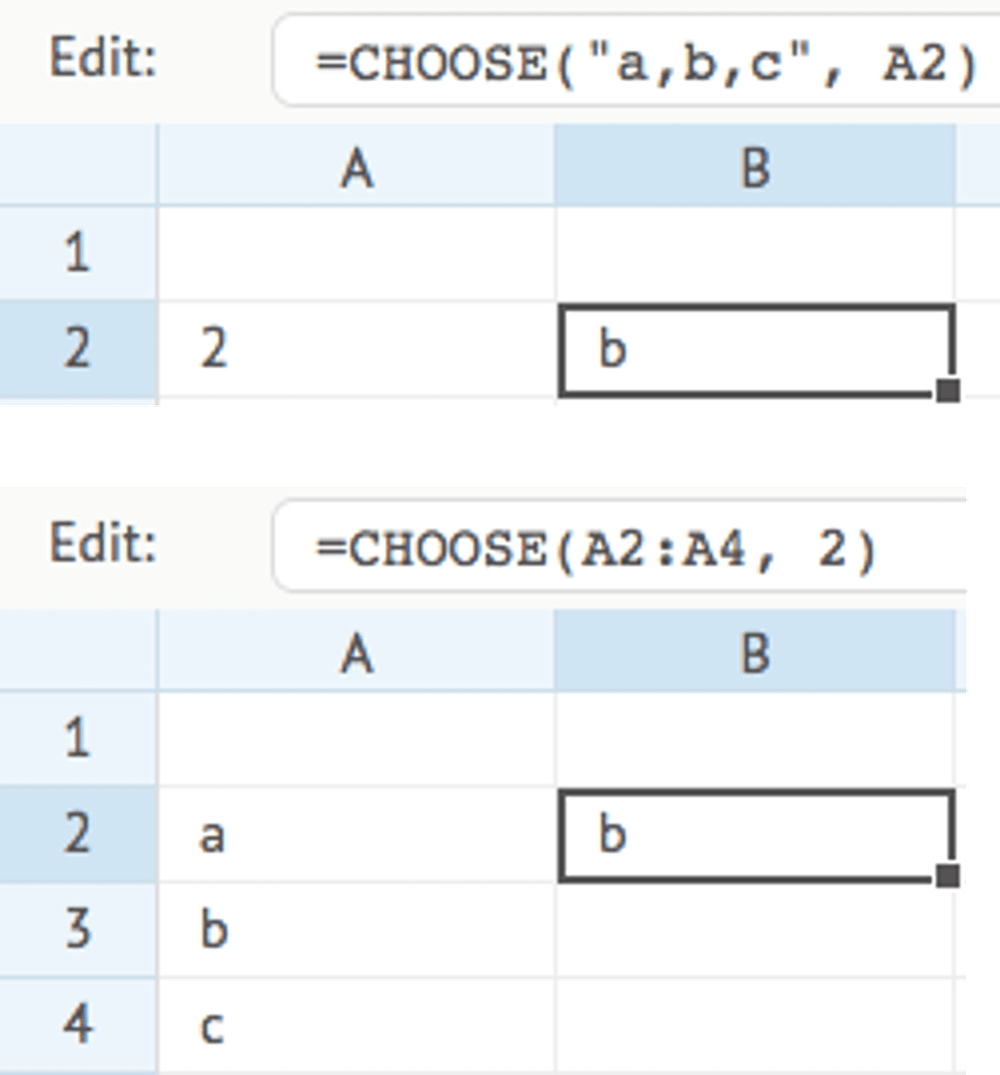#### COLUMNS

Returns the number of columns in the given cell range or data array. The range must contain only one row.

COLUMNS(data)

Example:
COLUMNS(A1:E1) = 5

#### COMPARE ❖ XLReporting

Compares the values in the 2 given cell ranges or data arrays, and returns the outcome defined by the type parameter. Both ranges need to be of comparable size. The common use-case for this function is to understand the difference in values between 2 ranges.

COMPARE(data, range, type)

Type:
0 = returns true if the values in both ranges are the same, else returns false (default)
1 = returns the number of values that differ between both ranges
2 = returns the changed values as a comma-delimited list
3 = returns the changed values and the compared values as a comma-delimited list

Example:
COMPARE(A1:A5, B1:B5, 3) = "Peter Smith (John Smith)"

#### DISTINCT ❖ XLReporting

Returns a sorted list with distinct items that occur in the given cell range, data array, or comma-delimited list of values. You can also use this function to ensure that reports and queries retrieve detailed data for each distinct value in this field, rather than aggregated.

DISTINCT(data)

Example:
data = "a,a,b,a,c,b,c,b,a"
DISTINCT(data) = "a,b,c"

#### EXIST ❖ XLReporting

Returns true if the given data equals any of the given values, else returns false. You can provide any number of values. Values can be single values or arrays or cell ranges. This function works with numbers, texts, dates, or periods.

EXIST(data, values, values, ..)

Example:
data = "C"
EXIST(data, "A", "B", "C", "D") = true

#### EXTRACT ❖ XLReporting

Returns the value or text that resides between the given key and endkey. This function is useful to extract words from texts, or to extract values from within HTML or XML tags.

EXTRACT(data, key, endkey)

Example:
data = "<product>coffee</product> "
EXTRACT(data, "<product>", "</product>") = "coffee"

#### FILTER

Returns a filtered list with items in the given cell range or data array that meet the given condition. The condition can either be a single value if you just want to compare against that value, or an expression with an operator and value, within quotes (e.g. ">10").

The data parameter is the range of which you want to return the filtered items that meet the condition. If you want to return the items of a range other than data, you can optionally provide the range parameter. You can omit range if it is the same as the data range. Using the optional sort parameter you can sort the list.

This function is similar to the well-known functions SUMIF and COUNTIF, but rather than returning the sum or count of matching items, it returns a filtered (and optionally sorted) list of items.

FILTER(data, condition, range, sort)

Example:
FILTER(A2:A9, ">1000") = "1100,2002,1090"
FILTER(A2:A9, ">1000", A2:A9, 1) = "1090,1100,2002"

#### FIND

Returns the starting position of a given text string (the key parameter) within another text string (the data parameter).

FIND(data, key)

Example:
data = "this text"
FIND(data, "tex") = 6

#### FIRSTVALUE ❖ XLReporting

Returns the first non-blank and non-zero value in the given cell range of data array. You may specific multiple ranges.

FIRSTVALUE(data, data, ..)

Example:
FIRSTVALUE("", "a", "", "b", "") = "a"

#### HLOOKUP

Looks up the given key value in the given horizontal cell range or data array (data), and returns the value in the same column in the second horizontal cell range or data array (range).

The lookup will always try an exact match on the key value, and return an empty text when no exact match is found. If you want to find the nearest or approximate match when no exact match is found, you need to set the type parameter.

HLOOKUP(data, range, key, type)

Type:
0 = exact match only (default)
1 = find the next value just after the given key
2 = find the previous value just before the given key
3 = case-insensitive fuzzy match by ignoring all characters other than A-Z and 0-9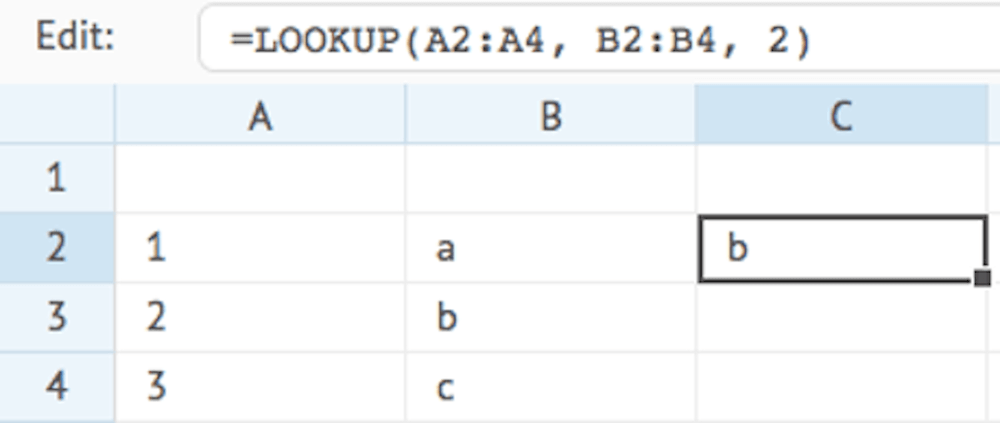#### INDEX

Returns a single value from the given cell range or data array, based on the given index. The index is a sequential result of the combined row and column position. For example, a range with 3 rows and 2 columns has 6 indices (3 * 2). The value on row 2 and column 1 has index 3 (1 * 2 + 1), whilst the value on row 3 and column 2 has index 6 (2 * 2 + 2), and so on.

INDEX(data, index)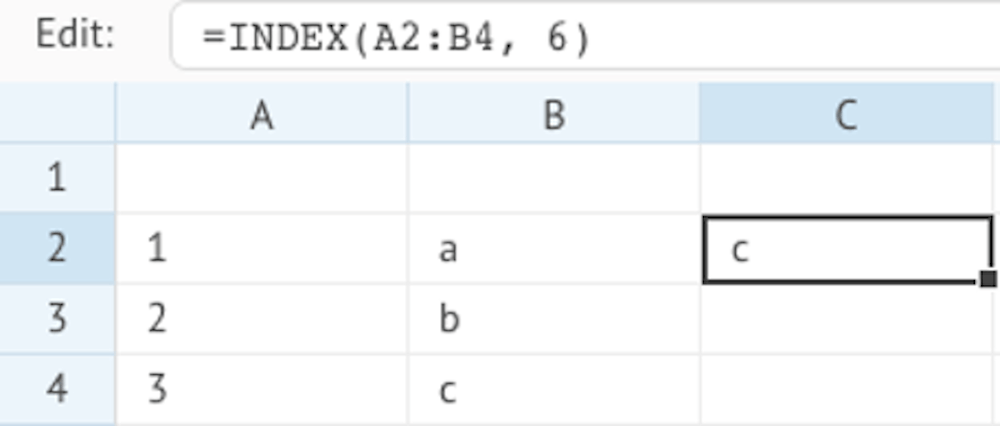#### INDIRECT

Returns the value in the cell with the given cell reference, or with the given row and column. You can optionally provide the sheet name (as text), else the default sheet (the first or only sheet in the model) will be used.

This is only relevant in models and enables you to indirectly obtain the value in a cell by setting a dynamic reference to that cell. This enables you to dynamically change the reference to a cell within a formula without having to change the formula itself.

Please be aware that sheet and cell references made via INDIRECT are not adjusted when sheets are renamed and/or rows or columns are inserted or deleted. INDIRECT enables you to set your own dynamic references to cell positions within a model, and is therefore never adjusted.

INDIRECT(cell) or INDIRECT(row, column, sheet)

Example:
INDIRECT("C2") = 25
INDIRECT(2, 3) = 25
INDIRECT(2, 3, "Sheet1") = 25

#### JSON ❖ XLReporting

Returns a property value from a JSON-formatted text, based on the given key. You can specify the nodes(s) that represent the property value you want. You can specify nested nodes by a period, e.g. mydata.product.description. You can also specify array positions, e.g. mydata.products.0.id

JSON(data, key)

Example:
data = {"mydata":{"product":{"id":"1","description":"coffee","price":"10"}}}
JSON(data, "mydata.product.description") = "coffee"

#### LABEL ❖ XLReporting

Returns a text label for the given index by looking it up in the given cell range, data array, or comma-delimited list of values.

LABEL(data, index)

Example:
LABEL("Jan,Feb,Mar", 2) = "Feb"

#### LASTVALUE ❖ XLReporting

Returns the last non-blank and non-zero value in the given cell range of data array. You may specific multiple ranges.

LASTVALUE(data, data, ..)

Example:
LASTVALUE("", "a", "", "b", "") = "b"

#### LOOKUP

Looks up the given key value in the given cell range or data array (data), and returns the value on the same row in the second cell range or data array (range).

The lookup will always try an exact match on the key value, and return an empty text when no exact match is found. If you want to find the nearest or approximate match when no exact match is found, you need to set the type parameter.

LOOKUP(data, range, key, type)

Type:
0 = exact match only (default)
1 = find the next value just after the given key
2 = find the previous value just before the given key
3 = case-insensitive fuzzy match by ignoring all characters other than A-Z and 0-9#### MATCH

Compares each item in the given cell range or data array (data) to a condition, and returns the value on the first row in the second cell range or data array (range) that matches the condition.

MATCH(data, range, condition)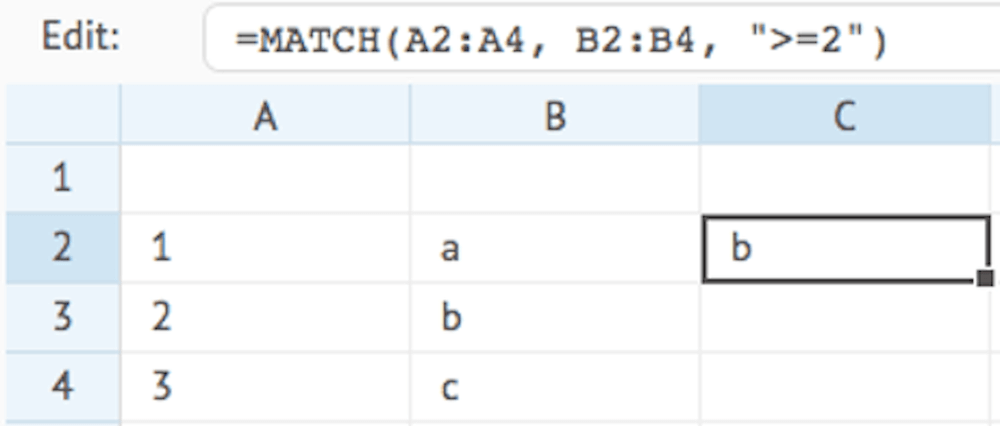#### OFFSET

Returns a single value from the given cell range or data array, based on the given index. The index is a sequential result of the combined row and column position. For example, a range with 3 rows and 2 columns has 6 indices (3 * 2). The value on row 2 and column 1 has index 3 (1 * 2 + 1), whilst the value on row 3 and column 2 has index 6 (2 * 2 + 2), and so on.

OFFSET(data, index)

Example:
OFFSET(A1:L1, 3) = the value in cell C1

#### PLAN ❖ XLReporting

Returns the range of values, or the value at the given index, of the given planner. See the PLANNER function below for further reference. No calculation is performed: the function simply returns the value(s), which you can use in your own formula.

If you omit index, the function returns a range with all values.

PLAN(planner, index)

Examples:
PLAN("Months", 6) = 0.0833  (1 / 12)

The PLAN function works in conjunction with the Cell editor Planner and enables you to define a pattern of values, and use those in formulas.

When you want to use a PLAN function, you first need to define a Cell editor Planner. See the PLANNER function for more details.

#### PLANNER ❖ XLReporting

Returns a proportion of data using the relative value at the given index of the given planner. The returned value is calculated as follows:

data * (value of given planner index / sum of all planner values)

If the given index does not exist in the planner, the function will use the given ratio (which can be a cell reference, a formula, or a given value) and will return the result of data * ratio.

If neither the index or a ratio exists, the function will return 0 (zero).

PLANNER(data, planner, index, ratio)

Examples:
PLANNER(1000, "Months", 6)     = 83.33  (1000 * 1 / 12)
PLANNER(1000, "Weeks445", 6)   = 96.15  (1000 * 5 / 52)
PLANNER(1000, "Summmer", 6)    = 111.11 (1000 * 4 / 36)
PLANNER(1000, "", 0, B3/C3)    = 200.00 (1000 * 1 / 10

The PLANNER function works in conjunction with the Cell editor Planner and enables you to distribute a given value across a range of cells in a model, based on a defined pattern that can be selected by the user. For example, this can be used to divide an given amount over a range of periods, or across products etc.

When you want to use a PLANNER function, you first need to define a Cell editor Planner.

The below example defines 4 patterns for a cell editor: monthly, quarterly, every half year, and a custom pattern: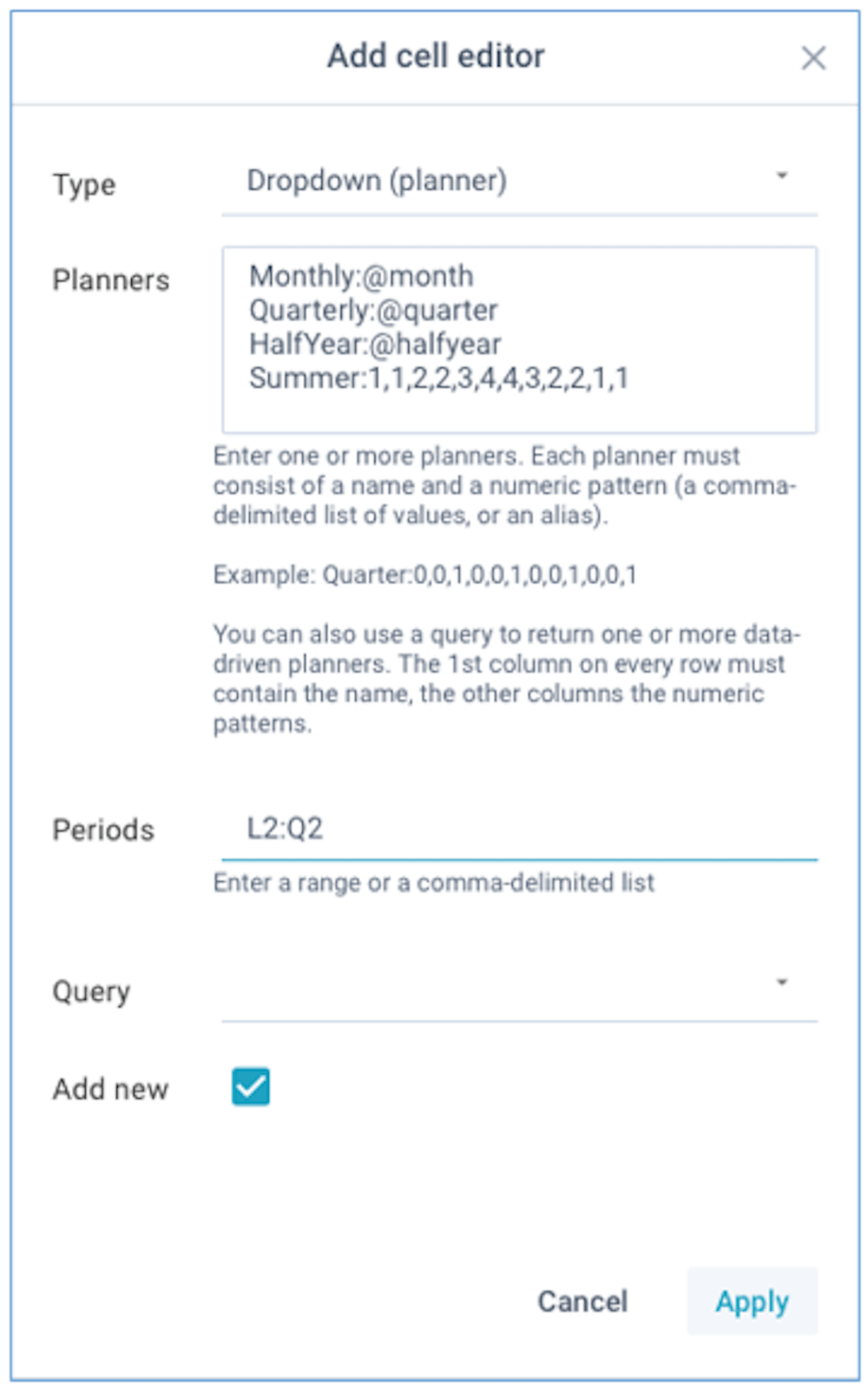The absolute values of the numeric patterns are irrelevant, what matters is each relative value as a proportion of the sum of all values. You can define as many indices in the patterns as you need.

You can also use one of the below aliases for common patterns:

• @month - every month (1,1,1,1,1,1,1,1,1,1,1,1)
• @quarter - every quarter (0,0,1,0,0,1,0,0,1,0,0,1)
• @soquarter - start of every quarter (1,0,0,1,0,0,1,0,0,1,0,0)
• @halfyear - every half year (0,0,0,0,0,1,0,0,0,0,0,1)
• @sohalfyear - start of every half year (1,0,0,0,0,0,1,0,0,0,0,0)
• @year - every year (0,0,0,0,0,0,0,0,0,0,0,1)
• @soyear - start of every year (1,0,0,0,0,0,0,0,0,0,0,0)
• @period445 - based on 4-4-5 weeks (4,4,5,4,4,5,4,4,5,4,4,5)

In addition to defined static planners, you can also refer to a data query. Your data query can include multiple rows, with each row being treated as a separate planner. The first column on every row needs to contain the planner name, and the other columns need to contain the numeric patterns.

❖ XLReporting

#### PRETTY

Returns a formatted and easily readable text from the given JSON-formatted text, cell range, data array, or comma-delimited list of values (data), each value prefixed with its associated labels. The labels have to be a comma-delimited list of values.

The result will be a text in the form of "label1=value1, label2=value2, ..." with each value joined with its respective label. Values without associated labels will be ignored.

PRETTY(data, labels)

Example:
PRETTY(A2:A3, "key,value") = "key=abc, value=1000"
PRETTY("abc,1000", "key,value") = "key=abc, value=1000"
PRETTY("{"a":"abc","b":"1000"}", "key,value") = "key=abc, value=1000"

#### RANK

Returns the rank (starting at 1) of a given number within the list of numbers in the given cell range or data array. Using the optional type parameter, you can decide if (and how) the list will be sorted.

RANK(data, number, type)

Type:
"asc"  = ascending sort (default)
"desc" = descending sort
"none" = no sort

Example:
RANK(A1:A4, 45, "asc") = 3

#### ROWS

Returns the number of rows in the given cell range or data array. The range must contain only one column.

ROWS(data)

Example:
ROWS(A1:A4) = 4

#### SEQUENCE

Returns an array of numbers, dates, or periods from the given start for the given number of increments. The returned data has the same structure as a cell range or data array, which enables you to pass this data directly into a dropdown editor or other functions that expect a cell range or data array. You can optionally pass an interval (default is 1). The interval can be positive or negative.

You can also use a text as data, in which case it will be suffixed by a numeric series.

This function automatically determines the type of data and then calls either PERIODRANGE, DATERANGE, or the NUMRANGE function.

SEQUENCE(data, number, interval)

Example:
SEQUENCE(5, 4) = 5, 6, 7, 8
SEQUENCE(5, 4, 10) = 5, 15, 25, 35
SEQUENCE("2019-01", 3, 12) = "2019-01", "2020-01", "2021-01"
SEQUENCE("col", 4) = "col0", "col1", "col2", "col3"

#### SETWRAP ❖ XLReporting

Updates a specific value within a JSON-formatted text that was created by WRAP. The key is numeric in order of the values passed to WRAP (e.g. 1, 2, 3 etc). Use WRAP to create the JSON-formatted text, or UNWRAP to extract the data again.

SETWRAP(data, value, key)

Example:
SETWRAP(data, 25, 2)

#### SWITCH

Returns the corresponding value that matches the given key in the given cell range or data array, or comma-delimited list of values. The list must contain the keys and their corresponding values as separate consequtive items. If no value is found, either the key is returned, or an empty value if you set the empty parameter to true.

SWITCH(data, key, empty)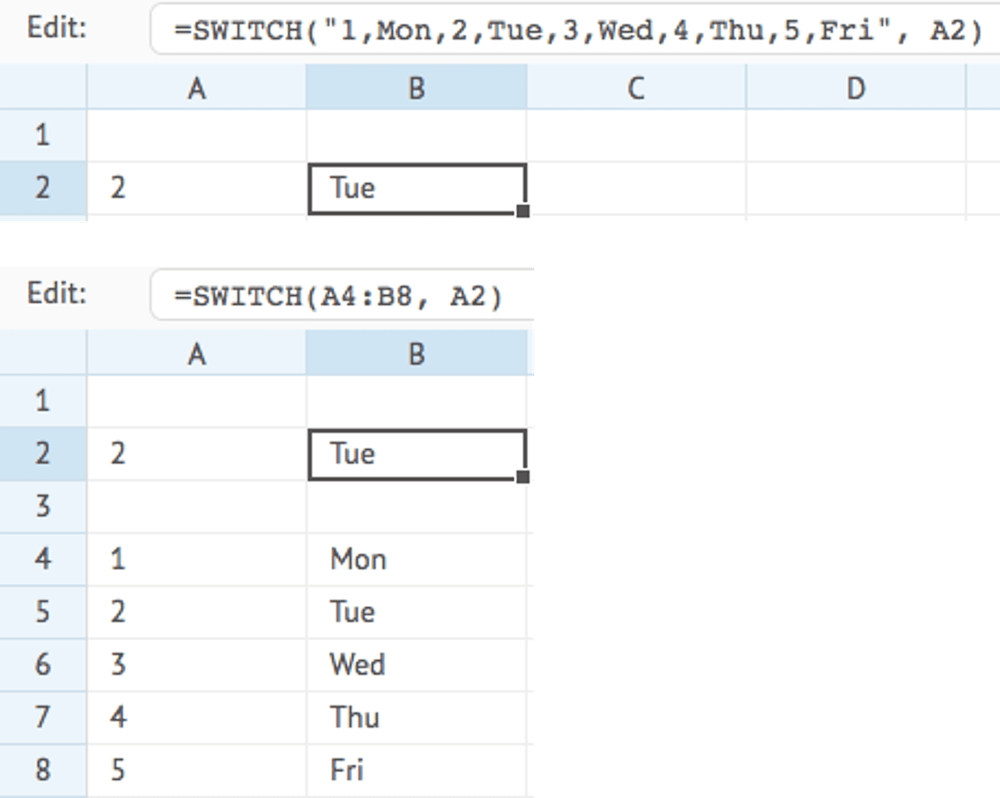#### TAKE ❖ XLReporting

Returns an array with the given cell values, arrays or cell ranges. This is an alias to CELLRANGE. Using INDEX you can extract individual values from the array. You may specific multiple ranges.

TAKE(data, data, ..)

Example:
TAKE(A1:A3, B5, C10:C12)

#### TYPE

Returns the type of value in the given data. If the optional type parameter is given, returns true if the data matches the expected type.

TYPE(data, type)

Type:
array   = a data array or cell range
blank   = the following values are considered blank: absent data, empty string "", a single space " ", a "0" text, and a 0 number
error   = a formula error
period  = a period YYYY-MM
date    = a date YYYY-MM-DD
number  = a numerical value
boolean = a boolean value
phone   = a phone number
url     = a website URL
wrap    = a JSON-formatted text (see WRAP function)
text    = a text string

Example:
TYPE(123) = "number"
TYPE(123, "number") = true
CELL(A3) = "text"

#### UNIQUE

Returns a sorted list with unique items that occur in the given cell range, data array, or comma-delimited list of values.

UNIQUE(data)

Example:
data = "a,a,b,a,c,b,c,b,a"
UNIQUE(data) = "a,b,c"

#### UNWRAP ❖ XLReporting

Returns a specific value from a JSON-formatted text that was created by WRAP. The key is numeric in order of the values passed to WRAP (e.g. 1, 2, 3 etc). Use WRAP to create the JSON-formatted text.

UNWRAP(data, key)

Example:
UNWRAP(data, 2)

#### VLOOKUP

Looks up the given key value in the given vertical cell range or data array, and returns the value on the same row in the second horizontal cell range or data array (range).

The lookup will always try an exact match on the key value, and return an empty text when no exact match is found. If you want to find the nearest or approximate match when no exact match is found, you need to set the type parameter.

VLOOKUP(data, range, key, type)

Type:
0 = exact match only (default)
1 = find the next value just after the given key
2 = find the previous value just before the given key
3 = case-insensitive fuzzy match by ignoring all characters other than A-Z and 0-9#### WEBSERVICE

Returns data from a web service on the Internet or Intranet. Only connections over https:// are allowed.

If the data is returned in JSON format, you can use the key parameter to specify the JSON node(s) that contains the required value(s). You can specify nested nodes by a period, e.g. mydata.product.description. You can also specify array positions, e.g. mydata.products..id

If you don't specify any node, the entire JSON data will be returned as text and you can use the JSON function to extract the necessary value(s) from that data.

If the data is returned in text, XML, or HTML format, you can use standard lookup and text functions (e.g. FIND, EXTRACT, LEFT, MID, RIGHT etc) to extract the required value(s).

WEBSERVICE(url, key)

Example:
WEBSERVICE("https://api.exchangeratesapi.io/latest", "rates.USD")

#### WEBVIEW ❖ XLReporting

Views the content of a web page on the Internet or Intranet. You can optionally define the width and height of the window that contains the content.

WEBVIEW(url, width, height)

Example:
WEBVIEW("https://www.xlreporting.com")

#### WITHIN ❖ XLReporting

Returns true if the given data falls between any of the given from/to value pairs, else returns false. You can provide any number of values. This function works with numbers, texts, dates, or periods.

WITHIN(data, from, to, from, to, ..)

Example:
data = 100
WITHIN(data, 120, 140, 80, 110, 30, 40) = true

#### WRAP ❖ XLReporting

Wraps a given cell range or data array into a JSON-formatted text. Ideal for storing complex data into a single value, for example when saving into a data set column. Each value is given a sequential numeric key starting at 1 (e.g. 1, 2, 3 etc) in order of the values passed. Use UNWRAP to extract the data again, or SETWRAP to update a specific value. You may specific multiple ranges.

WRAP(data, data, ..)

Example:
WRAP(A3:B3)
WRAP(A3, B3, C3)

#### XLOOKUP

Looks up the given key value in the given cell range or data array (data), and returns the value on the same row in the second cell range or data array (range).

The lookup will always try an exact match on the key value, and return an empty text (or the optional notfound value) when no exact match is found. If you want to find the nearest or approximate match when no exact match is found, you need to set the type parameter.

XLOOKUP(data, range, key, notfound, type)

Type:
0 = exact match only (default)
1 = find the next value just after the given key
2 = find the previous value just before the given key
3 = case-insensitive fuzzy match by ignoring all characters other than A-Z and 0-9

#### XMATCH

Looks up the given key value in the given cell range or data array (data), and returns its relative position.

The lookup will always try an exact match on the key value, and return an empty text when no exact match is found. If you want to find the nearest or approximate match when no exact match is found, you need to set the type parameter.

XMATCH(data, key, type)

Type:
0 = exact match only (default)
1 = find the next value just after the given key
2 = find the previous value just before the given key
3 = case-insensitive fuzzy match by ignoring all characters other than A-Z and 0-9